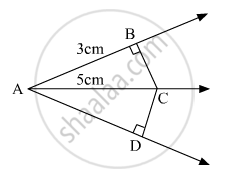# In the Given Figure, If Ac is Bisector of ∠Bad Such that Ab = 3 Cm and Ac = 5 Cm, Then Cd = - Mathematics

MCQ

In the given figure, if AC is bisector of ∠BAD such that AB = 3 cm and AC =  5 cm, then CD =•  2 cm

•  3 cm

•  4 cm

•  5 cm

#### Solution

It is given that

AB =  3cm

AC =5cm

We are to find the side CDAnalyze the figure and conclude that

CD = BC (As in the two triangles are congruent)

In ΔABC

BC = sqrt25- 9

= sqrt 16

= 4

So

BC = CD

⇒ CD = 4

Hence (c)  CD = 4.

Concept: Congruence of Triangles
Is there an error in this question or solution?

#### APPEARS IN

RD Sharma Mathematics for Class 9
Chapter 12 Congruent Triangles
Exercise 12.8 | Q 16 | Page 87
Share